## Resource Allocation Graph-

Before you go through this article, make sure that you have gone through the previous article on Resource Allocation Graph.

We have discussed-

• Resource Allocation Graph (RAG) is a graph that represents the state of a system pictorially.
• There are two components of RAG- Vertices and Edges.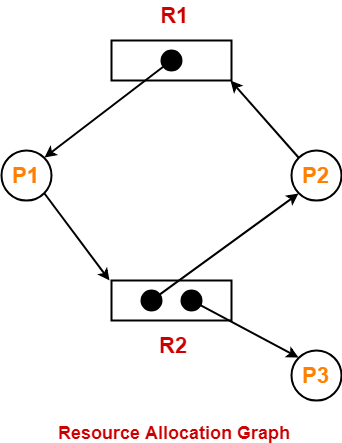Using Resource Allocation Graph, it can be easily detected whether system is in a Deadlock state or not.

The rules are-

## Rule-01:

In a Resource Allocation Graph where all the resources are single instance,

• If a cycle is being formed, then system is in a deadlock state.
• If no cycle is being formed, then system is not in a deadlock state.

## Rule-02:

In a Resource Allocation Graph where all the resources are NOT single instance,

• If a cycle is being formed, then system may be in a deadlock state.
• Banker’s Algorithm is applied to confirm whether system is in a deadlock state or not.
• If no cycle is being formed, then system is not in a deadlock state.
• Presence of a cycle is a necessary but not a sufficient condition for the occurrence of deadlock.

## Problem-01:

Consider the resource allocation graph in the figure-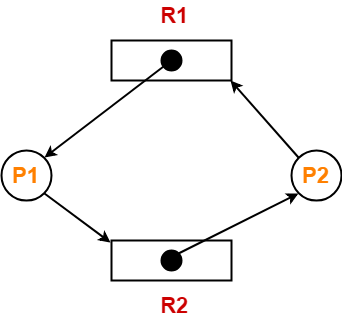Find if the system is in a deadlock state otherwise find a safe sequence.

## Solution-

### Method-01:

• The given resource allocation graph is single instance with a cycle contained in it.
• Thus, the system is definitely in a deadlock state.

### Method-02:

Using the given resource allocation graph, we have-

 Allocation Need R1 R2 R1 R2 Process P1 1 0 0 1 Process P2 0 1 1 0

Available = [ R1 R2 ] = [ 0 0 ]

Now,

• There are no instances available currently and both the processes require a resource to execute.
• Therefore, none of the process can be executed and both keeps waiting infinitely.
• Thus, the system is in a deadlock state.

## Problem-02:

Consider the resource allocation graph in the figure-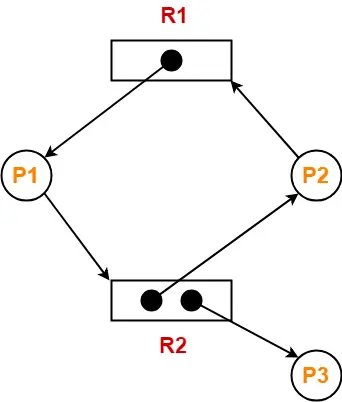Find if the system is in a deadlock state otherwise find a safe sequence.

## Solution-

• The given resource allocation graph is multi instance with a cycle contained in it.
• So, the system may or may not be in a deadlock state.

Using the given resource allocation graph, we have-

 Allocation Need R1 R2 R1 R2 Process P1 1 0 0 1 Process P2 0 1 1 0 Process P3 0 1 0 0

Available = [ R1 R2 ] = [ 0 0 ]

### Step-01:

• Since process P3 does not need any resource, so it executes.
• After execution, process P3 release its resources.

Then,

Available

= [ 0 0 ] + [ 0 1 ]

= [ 0 1 ]

### Step-02:

• With the instances available currently, only the requirement of the process P1 can be satisfied.
• So, process P1 is allocated the requested resources.
• It completes its execution and then free up the instances of resources held by it.

Then-

Available

= [ 0 1 ] + [ 1 0 ]

= [ 1 1 ]

### Step-03:

• With the instances available currently, the requirement of the process P2 can be satisfied.
• So, process P2 is allocated the requested resources.
• It completes its execution and then free up the instances of resources held by it.

Then-

Available

= [ 1 1 ] + [ 0 1 ]

= [ 1 2 ]

Thus,

• There exists a safe sequence P3, P1, P2 in which all the processes can be executed.
• So, the system is in a safe state.

## Problem-03:

Consider the resource allocation graph in the figure-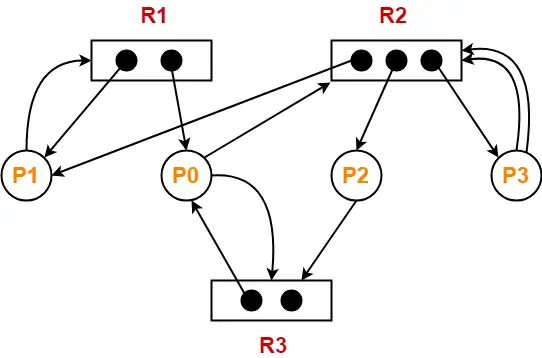Find if the system is in a deadlock state otherwise find a safe sequence.

## Solution-

• The given resource allocation graph is multi instance with a cycle contained in it.
• So, the system may or may not be in a deadlock state.

Using the given resource allocation graph, we have-

 Allocation Need R1 R2 R3 R1 R2 R3 Process P0 1 0 1 0 1 1 Process P1 1 1 0 1 0 0 Process P2 0 1 0 0 0 1 Process P3 0 1 0 0 2 0

Available = [ R1 R2 R3 ] = [ 0 0 1 ]

### Step-01:

• With the instances available currently, only the requirement of the process P2 can be satisfied.
• So, process P2 is allocated the requested resources.
• It completes its execution and then free up the instances of resources held by it.

Then-

Available

= [ 0 0 1 ] + [ 0 1 0 ]

= [ 0 1 1 ]

### Step-02:

• With the instances available currently, only the requirement of the process P0 can be satisfied.
• So, process P0 is allocated the requested resources.
• It completes its execution and then free up the instances of resources held by it.

Then-

Available

= [ 0 1 1 ] + [ 1 0 1 ]

= [ 1 1 2 ]

### Step-03:

• With the instances available currently, only the requirement of the process P1 can be satisfied.
• So, process P1 is allocated the requested resources.
• It completes its execution and then free up the instances of resources held by it.

Then-

Available

= [ 1 1 2 ] + [ 1 1 0 ]

= [ 2 2 2 ]

### Step-04:

• With the instances available currently, the requirement of the process P3 can be satisfied.
• So, process P3 is allocated the requested resources.
• It completes its execution and then free up the instances of resources held by it.

Then-

Available

= [ 2 2 2 ] + [ 0 1 0 ]

= [ 2 3 2 ]

Thus,

• There exists a safe sequence P2, P0, P1, P3 in which all the processes can be executed.
• So, the system is in a safe state.

To watch video solutions and practice other problems,

Watch this Video Lecture

Next Article- Contiguous Memory Allocation

Get more notes and other study material of Operating System.

Watch video lectures by visiting our YouTube channel LearnVidFun.

## Resource Allocation Graph-

 Resource Allocation Graph (RAG) is a graph that represents the state of a system pictorially.

It gives complete information about the state of a system such as-

• How many processes exist in the system?
• How many instances of each resource type exist?
• How many instances of each resource type are allocated?
• How many instances of each resource type are still available?
• How many instances of each resource type are held by each process?
• How many instances of each resource type does each process need for execution?

## Components Of RAG-

There are two major components of a Resource Allocation Graph-

1. Vertices
2. Edges

## Vertices-

There are following types of vertices in a Resource Allocation Graph-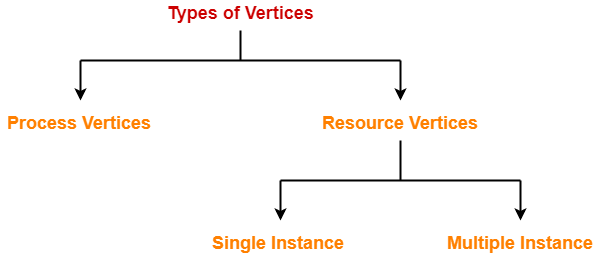1. Process Vertices
2. Resource Vertices

## Process Vertices-

• Process vertices represent the processes.
• They are drawn as a circle by mentioning the name of process inside the circle.

## Resource Vertices-

• Resource vertices represent the resources.
• Depending on the number of instances that exists in the system, resource vertices may be single instance or multiple instance.
• They are drawn as a rectangle by mentioning the dots inside the rectangle.
• The number of dots inside the rectangle indicates the number of instances of that resource existing in the system.

## Edges-

There are two types of edges in a Resource Allocation Graph-1. Assign Edges
2. Request Edges

## Assign Edges-

• Assign edges represent the assignment of resources to the processes.
• They are drawn as an arrow where the head of the arrow points to the process and tail of the process points to the instance of the resource.

## Request Edges-

• Request edges represent the waiting state of processes for the resources.
• They are drawn as an arrow where the head of the arrow points to the instance of the resource and tail of the process points to the process.
• If a process requires ‘n’ instances of a resource type, then ‘n’ assign edges will be drawn.

## Example Of RAG-

The following diagram represents a Resource Allocation Graph-It gives the following information-

• There exist three processes in the system namely P1, P2 and P3.
• There exist two resources in the system namely R1 and R2.
• There exists a single instance of resource R1 and two instances of resource R2.
• Process P1 holds one instance of resource R1 and is waiting for an instance of resource R2.
• Process P2 holds one instance of resource R2 and is waiting for an instance of resource R1.
• Process P3 holds one instance of resource R2 and is not waiting for anything.

To gain better understanding about Resource Allocation Graph,

Watch this Video Lecture

Next Article- Practice Problems On Resource Allocation Graph

Get more notes and other study material of Operating System.

Watch video lectures by visiting our YouTube channel LearnVidFun.

## Banker’s Algorithm-

Before you go through this article, make sure that you gone through the previous article on Banker’s Algorithm.

We have discussed-

• Banker’s Algorithm is a deadlock avoidance algorithm.
• It maintains a set of data using which it decides whether to entertain the request of any process or not.
• It follows the safety algorithm to check whether the system is in a safe state or not.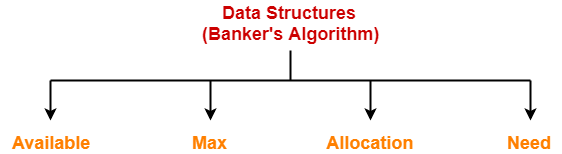## Problem-01:

A single processor system has three resource types X, Y and Z, which are shared by three processes. There are 5 units of each resource type. Consider the following scenario, where the column alloc denotes the number of units of each resource type allocated to each process, and the column request denotes the number of units of each resource type requested by a process in order to complete execution. Which of these processes will finish LAST?

1. P0
2. P1
3. P2
4. None of the above since the system is in a deadlock

 Alloc Request X Y Z X Y Z P0 1 2 1 1 0 3 P1 2 0 1 0 1 2 P2 2 2 1 1 2 0

## Solution-

According to question-

• Total = [ X Y Z ] = [ 5 5 5 ]
• Total _Alloc = [ X Y Z ] = [5 4 3]

Now,

Available

= Total – Total_Alloc

= [ 5 5 5 ] – [5 4 3]

= [ 0 1 2 ]

### Step-01:

• With the instances available currently, only the requirement of the process P1 can be satisfied.
• So, process P1 is allocated the requested resources.
• It completes its execution and then free up the instances of resources held by it.

Then,

Available

= [ 0 1 2 ] + [ 2 0 1]

= [ 2 1 3 ]

### Step-02:

• With the instances available currently, only the requirement of the process P0 can be satisfied.
• So, process P0 is allocated the requested resources.
• It completes its execution and then free up the instances of resources held by it.

Then-

Available

= [ 2 1 3 ] + [ 1 2 1 ]

= [ 3 3 4 ]

### Step-03:

• With the instances available currently, the requirement of the process P2 can be satisfied.
• So, process P2 is allocated the requested resources.
• It completes its execution and then free up the instances of resources held by it.

Then-

Available

= [ 3 3 4 ] + [ 2 2 1 ]

= [ 5 5 5 ]

Thus,

• There exists a safe sequence P1, P0, P2 in which all the processes can be executed.
• So, the system is in a safe state.
• Process P2 will be executed at last.

Thus, Option (C) is correct.

## Problem-02:

An operating system uses the banker’s algorithm for deadlock avoidance when managing the allocation of three resource types X, Y and Z to three processes P0, P1 and P2. The table given below presents the current system state. Here, the Allocation matrix shows the current number of resources of each type allocated to each process and the Max matrix shows the maximum number of resources of each type required by each process during its execution.

 Allocation Max X Y Z X Y Z P0 0 0 1 8 4 3 P1 3 2 0 6 2 0 P2 2 1 1 3 3 3

There are 3 units of type X, 2 units of type Y and 2 units of type Z still available. The system is currently in safe state. Consider the following independent requests for additional resources in the current state-

REQ1: P0 requests 0 units of X, 0 units of Y and 2 units of Z

REQ2: P1 requests 2 units of X, 0 units of Y and 0 units of Z

Which of the following is TRUE?

1. Only REQ1 can be permitted
2. Only REQ2 can be permitted
3. Both REQ1 and REQ2 can be permitted
4. Neither REQ1 nor REQ2 can be permitted

## Solution-

According to question,

Available = [ X Y Z ] = [ 3 2 2 ]

Now,

Need = Max – Allocation

So, we have-

 Allocation Max Need X Y Z X Y Z X Y Z P0 0 0 1 8 4 3 8 4 2 P1 3 2 0 6 2 0 3 0 0 P2 2 1 1 3 3 3 1 2 2

Currently, the system is in safe state.

(It is given in question. If we want, we can check)

### Checking Whether REQ1 Can Be Entertained-

• Need of P0 = [ 0 0 2 ]
• Available = [ 3 2 2 ]

Clearly,

• With the instances available currently, the requirement of REQ1 can be satisfied.
• So, banker’s algorithm assumes that the request REQ1 is entertained.
• It then modifies its data structures as-

 Allocation Max Need X Y Z X Y Z X Y Z P0 0 0 3 8 4 3 8 4 0 P1 3 2 0 6 2 0 3 0 0 P2 2 1 1 3 3 3 1 2 2

Available

= [ 3 2 2 ] – [ 0 0 2 ]

= [ 3 2 0 ]

• Now, it follows the safety algorithm to check whether this resulting state is a safe state or not.
• If it is a safe state, then REQ1 can be permitted otherwise not.

### Step-01:

• With the instances available currently, only the requirement of the process P1 can be satisfied.
• So, process P1 is allocated the requested resources.
• It completes its execution and then free up the instances of resources held by it.

Then-

Available

= [ 3 2 0 ] + [ 3 2 0 ]

= [ 6 4 0 ]

Now,

• It is not possible to entertain any process.
• The system has entered the deadlock state which is an unsafe state.
• Thus, REQ1 will not be permitted.

### Checking Whether REQ2 Can Be Entertained-

• Need of P1 = [ 2 0 0 ]
• Available = [ 3 2 2 ]

Clearly,

• With the instances available currently, the requirement of REQ1 can be satisfied.
• So, banker’s algorithm assumes the request REQ2 is entertained.
• It then modifies its data structures as-

 Allocation Max Need X Y Z X Y Z X Y Z P0 0 0 1 8 4 3 8 4 2 P1 5 2 0 6 2 0 1 0 0 P2 2 1 1 3 3 3 1 2 2

Available

= [ 3 2 2 ] – [ 2 0 0 ]

= [ 1 2 2 ]

• Now, it follows the safety algorithm to check whether this resulting state is a safe state or not.
• If it is a safe state, then REQ2 can be permitted otherwise not.

### Step-01:

• With the instances available currently, only the requirement of the process P1 can be satisfied.
• So, process P1 is allocated the requested resources.
• It completes its execution and then free up the instances of resources held by it.

Then-

Available

= [ 1 2 2 ] + [ 5 2 0 ]

= [ 6 4 2 ]

### Step-02:

• With the instances available currently, only the requirement of the process P2 can be satisfied.
• So, process P2 is allocated the requested resources.
• It completes its execution and then free up the instances of resources held by it.

Then-

Available

= [ 6 4 2 ] + [ 2 1 1 ]

= [ 8 5 3 ]

### Step-03:

• With the instances available currently, the requirement of the process P0 can be satisfied.
• So, process P0 is allocated the requested resources.
• It completes its execution and then free up the instances of resources held by it.

Then-

Available

= [ 8 5 3 ] + [ 0 0 1 ]

= [ 8 5 4 ]

Thus,

• There exists a safe sequence P1, P2, P0 in which all the processes can be executed.
• So, the system is in a safe state.
• Thus, REQ2 can be permitted.

Thus, Correct Option is (B).

## Problem-03:

A system has 4 processes and 5 allocatable resource. The current allocation and maximum needs are as follows-

 Allocated Maximum A 1 0 2 1 1 1 1 2 1 3 B 2 0 1 1 0 2 2 2 1 0 C 1 1 0 1 1 2 1 3 1 1 D 1 1 1 1 0 1 1 2 2 0

If Available = [ 0 0 X 1 1 ], what is the smallest value of x for which this is a safe state?

## Solution-

Let us calculate the additional instances of each resource type needed by each process.

We know,

Need = Maximum – Allocation

So, we have-

 Need A 0 1 0 0 2 B 0 2 1 0 0 C 1 0 3 0 0 D 0 0 1 1 0

### Case-01: For X = 0

If X = 0, then-

Available

= [ 0 0 0 1 1 ]

• With the instances available currently, the requirement of any process can not be satisfied.
• So, for X = 0, system remains in a deadlock which is an unsafe state.

If X = 1, then-

Available

= [ 0 0 1 1 1 ]

### Step-01:

• With the instances available currently, only the requirement of the process D can be satisfied.
• So, process D is allocated the requested resources.
• It completes its execution and then free up the instances of resources held by it.

Then-

Available

= [ 0 0 1 1 1 ] + [ 1 1 1 1 0 ]

= [ 1 1 2 2 1 ]

• With the instances available currently, the requirement of any process can not be satisfied.
• So, for X = 1, system remains in a deadlock which is an unsafe state.

If X = 2, then-

Available

= [ 0 0 2 1 1 ]

### Step-01:

• With the instances available currently, only the requirement of the process D can be satisfied.
• So, process D is allocated the requested resources.
• It completes its execution and then free up the instances of resources held by it.

Then-

Available

= [ 0 0 2 1 1 ] + [ 1 1 1 1 0 ]

= [ 1 1 3 2 1 ]

### Step-02:

• With the instances available currently, only the requirement of the process C can be satisfied.
• So, process C is allocated the requested resources.
• It completes its execution and then free up the instances of resources held by it.

Then-

Available

= [ 1 1 3 2 1 ] + [ 1 1 0 1 1 ]

= [ 2 2 3 3 2 ]

### Step-03:

• With the instances available currently, the requirement of both the processes A and B can be satisfied.
• So, processes A and B are allocated the requested resources one by one.
• They complete their execution and then free up the instances of resources held by it.

Then-

Available

= [ 2 2 3 3 2 ] + [ 1 0 2 1 1 ] + [ 2 0 1 1 0 ]

= [ 5 2 6 5 3 ]

Thus,

• There exists a safe sequence in which all the processes can be executed.
• So, the system is in a safe state.
• Thus, minimum value of X that ensures system is in safe state = 2.

To watch video solutions and practice other problems,

Watch this Video Lecture

Next Article- Resource Allocation Graph

Get more notes and other study material of Operating System.

Watch video lectures by visiting our YouTube channel LearnVidFun.

## Banker’s Algorithm | Deadlock Avoidance

Before you go through this article, make sure that you have gone through the previous article on Deadlock and Handling Strategies.

We have discussed-

• In a deadlock state, the execution of multiple processes is blocked.
• Deadlock avoidance strategy involves maintaining a set of data.
• The data is used to make a decision whether to entertain any new request or not.
• If entertaining the new request causes the system to move in an unsafe state, then it is discarded.

## Banker’s Algorithm-

• Banker’s Algorithm is a deadlock avoidance strategy.
• It is called so because it is used in banking systems to decide whether a loan can be granted or not.

## Prerequisite-

Banker’s Algorithm requires-

• Whenever a new process is created, it specifies the maximum number of instances of each resource type that it exactly needs.

## Data Structures Used-

To implement banker’s algorithm, following four data structures are used-1. Available
2. Max
3. Allocation
4. Need

 Data Structure Definition Example Available It is a single dimensional array that specifies the number of instances of each resource type currently available. Available[R1] = KIt means K instances of resource type R1 are currently available. Max It is a two dimensional array that specifies the maximum number of instances of each resource type that a process can request. Max[P1][R1] = KIt means process P1 is allowed to ask for maximum K instances of resource type R1. Allocation It is a two dimensional array that specifies the number of instances of each resource type that has been allocated to the process. Allocation[P1][R1] = KIt means K instances of resource type R1 have been allocated to the process P1. Need It is a two dimensional array that specifies the number of instances of each resource type that a process requires for execution. Need[P1][R1] = KIt means process P1 requires K more instances of resource type R1 for execution.

## Working-

• Banker’s Algorithm is executed whenever any process puts forward the request for allocating the resources.
• It involves the following steps-

### Step-01:

• Banker’s Algorithm checks whether the request made by the process is valid or not.
• If the request is invalid, it aborts the request.
• If the request is valid, it follows step-02.

### Valid Request

A request is considered valid if and only if-

The number of requested instances of each resource type is less than the need declared by the process in the beginning.

### Step-02:

• Banker’s Algorithm checks if the number of requested instances of each resource type is less than the number of available instances of each type.
• If the sufficient number of instances are not available, it asks the process to wait longer.
• If the sufficient number of instances are available, it follows step-03.

### Step-03:

• Banker’s Algorithm makes an assumption that the requested resources have been allocated to the process.
• Then, it modifies its data structures accordingly and moves from one state to the other state.

```Available = Available - Request(i)
Allocation(i) = Allocation(i) + Request(i)
Need(i) = Need(i) - Request(i)```

• Now, Banker’s Algorithm follows the safety algorithm to check whether the resulting state it has entered in is a safe state or not.
• If it is a safe state, then it allocates the requested resources to the process in actual.
• If it is an unsafe state, then it rollbacks to its previous state and asks the process to wait longer.

### Safe State

A system is said to be in safe state when-

All the processes can be executed in some arbitrary sequence with the available number of resources.

## Safety Algorithm Data Structures-

To implement safety algorithm, following two data structures are used-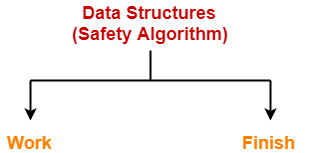1. Work
2. Finish

 Data Structure Definition Example Work It is a single dimensional array that specifies the number of instances of each resource type currently available. Work[R1] = KIt means K instances of resource type R1 are currently available. Finish It is a single dimensional array that specifies whether the process has finished its execution or not. Finish[P1] = 0It means process P1 is still left to execute.

## Safety Algorithm-

Safety Algorithm is executed to check whether the resultant state after allocating the resources is safe or not.

### Step-01:

Initially-

• Number of instances of each resource type currently available = Available
• All the processes are to be executed.
• So, in Step-01, the data structures are initialized as-

```Work = Available
Finish(i) = False for i = 0, 1, 2, ..., n-1```

### Step-02:

• Safety Algorithm looks for an unfinished process whose need is less than or equal to work.
• So, Step-02 finds an index i such that-

```Finish[ i ] = False
Need(i) <= Work.```

• If such a process exists, then step-03 is followed otherwise step-05 is followed.

### Step-03:

• After finding the required process, safety algorithm assumes that the requested resources are allocated to the process.
• The process runs, finishes its execution and the resources allocated to it gets free.
• The resources are then added to the work and finish(i) of that process is set as true.

```Work = Work + Allocation
Finish(i) = True```

### Step-04:

• The loop of Step-02 and Step-03 is repeated.

### Step-05:

• If all the processes can be executed in some sequence, then the system is said to be in a safe state.
• In other words, if Finish(i) becomes True for all i, then the system is in a safe state otherwise not.

To gain better understanding about Banker’s Algorithm,

Watch this Video Lecture

Next Article- Practice Problems On Banker’s Algorithm

Get more notes and other study material of Operating System.

Watch video lectures by visiting our YouTube channel LearnVidFun.

Before you go through this article, make sure that you have gone through the previous article on Deadlock in OS.

We have discussed-

• In a deadlock state, the execution of multiple processes is blocked.
• There are 4 necessary conditions for the occurrence of deadlock.

## Important Concept-

Consider there are n processes in the system P1, P2, P3, …… , Pn where-

• Process P1 requires xunits of resource R
• Process P2 requires xunits of resource R
• Process P3 requires xunits of resource R and so on.

In worst case,

 The number of units that each process holds = One less than its maximum demand

So,

• Process P1 holds x1 – 1 units of resource R
• Process P2 holds x– 1 units of resource R
• Process P3 holds x– 1 units of resource R and so on.

Now,

• Had there been one more unit of resource R in the system, system could be ensured deadlock free.
• This is because that unit would be allocated to one of the processes and it would get execute and then release its units.

From here, we have-

### Maximum Number Of Units That Ensures Deadlock-

Maximum number of units of resource R that ensures deadlock

= (x1-1) + (x2-1) + (x3-1) + …. + (xn-1)

= ( x1 + x2 + x3 + …. + xn ) – n

= ∑xi – n

= Sum of max needs of all n processes – n

### Minimum Number Of Units That Ensures No Deadlock-

Minimum number of units of resource R that ensures no deadlock

= One more than maximum number of units of resource R that ensures deadlock

= (∑xi – n) + 1

## Problem-01:

A system is having 3 user processes each requiring 2 units of resource R. The minimum number of units of R such that no deadlock will occur-

1. 3
2. 5
3. 4
4. 6

## Solution-

In worst case,

The number of units that each process holds = One less than its maximum demand

So,

• Process P1 holds 1 unit of resource R
• Process P2 holds 1 unit of resource R
• Process P3 holds 1 unit of resource R

Thus,

• Maximum number of units of resource R that ensures deadlock = 1 + 1 + 1 = 3
• Minimum number of units of resource R that ensures no deadlock = 3 + 1 = 4

## Problem-02:

A system is having 10 user processes each requiring 3 units of resource R. The minimum number of units of R such that no deadlock will occur _____?

## Solution-

In worst case,

The number of units that each process holds = One less than its maximum demand

So,

• Process P1 holds 2 units of resource R
• Process P2 holds 2 units of resource R
• Process P3 holds 2 units of resource R and so on.
• Process P10 holds 2 units of resource R

Thus,

• Maximum number of units of resource R that ensures deadlock = 10 x 2 = 20
• Minimum number of units of resource R that ensures no deadlock = 20 + 1 = 21

## Problem-03:

A system is having 3 user processes P1, P2 and P3 where P1 requires 2 units of resource R, P2 requires 3 units of resource R, P3 requires 4 units of resource R. The minimum number of units of R that ensures no deadlock is _____?

## Solution-

In worst case,

The number of units that each process holds = One less than its maximum demand

So,

• Process P1 holds 1 unit of resource R
• Process P2 holds 2 units of resource R
• Process P3 holds 3 units of resource R

Thus,

• Maximum number of units of resource R that ensures deadlock = 1 + 2 + 3 = 6
• Minimum number of units of resource R that ensures no deadlock = 6 + 1 = 7

## Problem-04:

A system is having 3 user processes P1, P2 and P3 where P1 requires 21 units of resource R, P2 requires 31 units of resource R, P3 requires 41 units of resource R. The minimum number of units of R that ensures no deadlock is _____?

## Solution-

In worst case,

The number of units that each process holds = One less than its maximum demand

So,

• Process P1 holds 20 units of resource R
• Process P2 holds 30 units of resource R
• Process P3 holds 40 units of resource R

Thus,

• Maximum number of units of resource R that ensures deadlock = 20 + 30 + 40 = 90
• Minimum number of units of resource R that ensures no deadlock = 90 + 1 = 91

## Problem-05:

If there are 6 units of resource R in the system and each process in the system requires 2 units of resource R, then how many processes can be present at maximum so that no deadlock will occur?

## Solution-

In worst case,

The number of units that each process holds = One less than its maximum demand

So,

• Process P1 holds 1 unit of resource R
• Process P2 holds 1 unit of resource R
• Process P3 holds 1 unit of resource R
• Process P4 holds 1 unit of resource R
• Process P5 holds 1 unit of resource R
• Process P6 holds 1 unit of resource R

Thus,

• Minimum number of processes that ensures deadlock = 6
• Maximum number of processes that ensures no deadlock = 6 – 1 = 5

## Problem-06:

If there are 6 units of resource R in the system and each process in the system requires 3 units of resource R, then how many processes can be present at maximum so that no deadlock will occur?

## Solution-

In worst case,

The number of units that each process holds = One less than its maximum demand

So,

• Process P1 holds 2 units of resource R
• Process P2 holds 2 units of resource R
• Process P3 holds 2 units of resource R

Thus,

• Minimum number of processes that ensures deadlock = 3
• Maximum number of processes that ensures no deadlock = 3 – 1 = 2

## Problem-07:

If there are 100 units of resource R in the system and each process in the system requires 2 units of resource R, then how many processes can be present at maximum so that no deadlock will occur?

## Solution-

In worst case,

The number of units that each process holds = One less than its maximum demand

So,

• Process P1 holds 1 unit of resource R
• Process P2 holds 1 unit of resource R
• Process P3 holds 1 unit of resource R and so on.
• Process P100 holds 1 unit of resource R

Thus,

• Minimum number of processes that ensures deadlock = 100
• Maximum number of processes that ensures no deadlock = 100 – 1 = 99

## Problem-08:

If there are 100 units of resource R in the system and each process in the system requires 4 units of resource R, then how many processes can be present at maximum so that no deadlock will occur?

## Solution-

In worst case,

The number of units that each process holds = One less than its maximum demand

So,

• Process P1 holds 3 units of resource R
• Process P2 holds 3 units of resource R
• Process P3 holds 3 units of resource R and so on.
• Process P33 holds 3 units of resource R
• Process P34 holds 1 unit of resource R

Thus,

• Minimum number of processes that ensures deadlock = 34
• Maximum number of processes that ensures no deadlock = 34 – 1 = 33

## Problem-09:

If there are 100 units of resource R in the system and each process in the system requires 5 units of resource R, then how many processes can be present at maximum so that no deadlock will occur?

## Solution-

In worst case,

The number of units that each process holds = One less than its maximum demand

So,

• Process P1 holds 4 units of resource R
• Process P2 holds 4 units of resource R
• Process P3 holds 4 units of resource R and so on.
• Process P25 holds 4 units of resource R

Thus,

• Minimum number of processes that ensures deadlock = 25
• Maximum number of processes that ensures no deadlock = 25 – 1 = 24

## Problem-10:

A computer system has 6 tape drives with n processes competing for them. Each process needs 3 tape drives. The maximum value of n for which the system is guaranteed to be deadlock free-

1. 2
2. 3
3. 4
4. 1

## Solution-

In worst case,

The number of tape drives that each process holds = One less than its maximum demand

So,

• Process P1 holds 2 tape drives
• Process P2 holds 2 tape drives
• Process P3 holds 2 tape drives

Thus,

• Minimum number of processes that ensures deadlock = 3
• Maximum number of processes that ensures no deadlock = 3 – 1 = 2

## Problem-11:

Consider a system having m resources of the same type. These resources are shared by 3 processes A, B and C which have peak demands of 3, 4 and 6 respectively. For what value of m, deadlock will not occur?

1. 7
2. 9
3. 10
4. 13

## Solution-

In worst case,

The number of units that each process holds = One less than its maximum demand

So,

• Process A holds 2 units of resource R
• Process B holds 3 units of resource R
• Process C holds 5 units of resource R

Thus,

• Maximum number of units of resource R that ensures deadlock = 2 + 3 + 5 = 10
• Minimum number of units of resource R that ensures no deadlock = 10 + 1 = 11

So, any number of units greater than 11 will ensure no deadlock.

Thus, Option (D) is correct.

## Problem-12:

Consider a system having m resources of the same type being shared by n processes. Resources can be requested and released by processes only one at a time. The system is deadlock free if and only if-

1. The sum of all max needs is < m+n
2. The sum of all max needs is > m+n
3. Both of above
4. None of these

## Solution-

We have derived above-

Maximum number of units of resource R that ensures deadlock = (∑xi – n)

Number of units of resource R must be > (∑xi – n)

i.e. m > (∑xi – n)

or ∑xi < m + n

Thus, Correct Option is (A).

## Problem-13:

Consider the following snapshot of a system running n processes. Process i is holding xi instances of a resource R for 1<=i<=n. Currently, all instances of R are occupied. Further, for all i, process i has placed a request for an additional yi instances while holding the xi instances it already has. There are exactly two processes p and q such that yp = yq = 0. Which of the following can serve as a necessary condition to guarantee that the system is not approaching a deadlock?

1. min(xp, xq) < maxk≠p,qyk
2. xp + xq >= mink≠p,qyk
3. min(xp, xq) < 1
4. min(xp, xq) >1

## Solution-

According to question, we have-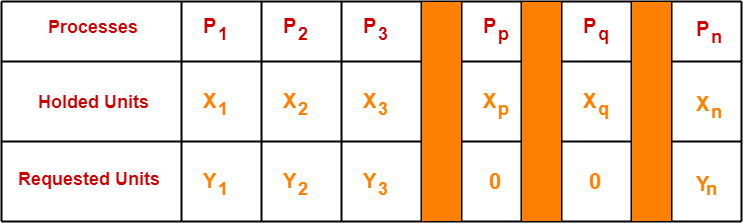• Clearly, processes Pp and Pq do not require any additional resource.
• So they continue their execution.
• After getting executed completely, they release the units allocated to them.
• Thus, the total units that get free up = xp + xq

Now,

• To ensure that other processes are executed without any deadlock, the total amount of units freely available currently ( xp + xq ) must be able to meet the requirements of some other process.
• If available ( xp + x) units could not meet the requirement of any other process, then certainly there would be deadlock.

Thus, for no deadlock, the necessary condition is-

xp + xq >= min yk where k ≠ p, q

Thus, Correct Option is (B).

### NOTE-

• It is very important to note that the above condition is just a necessary condition and not at all a sufficient condition to avoid the deadlock.
• The above condition just ensures that the system is able to proceed from the current state.
• It does not guarantee that there won’t be a deadlock before all the other processes are finished.
• The sufficient condition to avoid the deadlock would be either xp + xq >= ∑ yi or xp + xq >= max yk where k ≠ p, q.

To watch video solutions and practice other problems,

Watch this Video Lecture

Next Article- Banker’s Algorithm

Get more notes and other study material of Operating System.

Watch video lectures by visiting our YouTube channel LearnVidFun.